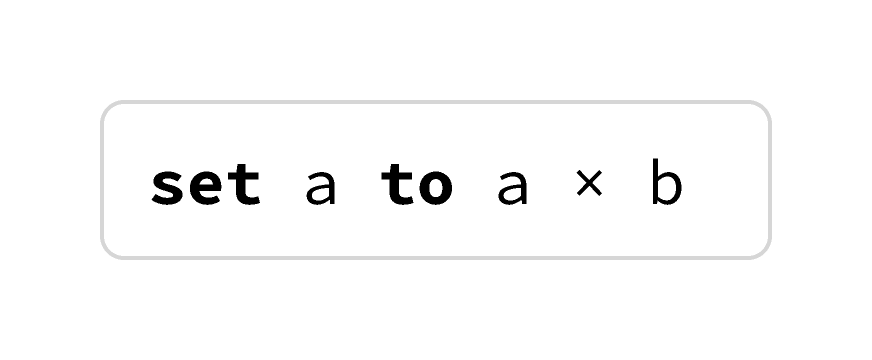### Algorithm Fundamentals

You’ve seen commands and conditionals. The last important component of any algorithmic language is repetition.

Your meticulous hamster sitter isn’t great with big numbers. Here’s an algorithm with repetition that you could give for feeding your particularly hungry hamster, Hugo:

Empty Hugo's bowl, and then straighten out the five fingers on your left hand.

Then, repeat the following two steps until there aren’t any more fingers spread out on your left hand. First, add a number of food pellets to Hugo's bowl equal to the number of uncurled fingers on your left hand. Second, curl up one finger.

How many food pellets will be in Hugo's bowl after your pet sitter runs this algorithm?# Repetition

Computer scientists like to use the word "while" when they describe repetition. For someone who has written a lot of algorithms or a lot of computer code, this description:

While you have an uncurled finger, put a pellet in the bowl and curl a finger.

means the same thing as this:

Repeat the following two steps as long as you still have an uncurled finger. First, put a pellet in the bowl. Second, curl a finger.

Maybe this use of the word "while" makes intuitive sense to you, and maybe it doesn't! Either way, there’s no need to reprogram the way you think about English. That’s one reason computer scientists describe algorithms with pseudocode instead of describing algorithms with paragraphs of text.

# Repetition

In code and pseudocode, when the word "while" is followed by some test, it is describing an algorithm that must

• run the test, and if the test succeeds, run the sequence of instructions underneath;
• then re-run the test, and if the test succeeds, run the sequence of instructions underneath (again);
• then re-run the test, and if the test succeeds, run the sequence of instructions underneath (again);
• ......;
• then re-run the test, and if the test fails, move on past the while instruction.

Here's what the Hugo-feeding algorithm looks like with a while instruction:

# Repetition

Rephrase this algorithm as an algorithm that uses numbers, assignables, and arithmetic. The number of pellets in the bowl should correspond to the value in the assignable n_pellets.

You won't use every line! Build the algorithm inside the dotted lines, and leave unused lines in the bank below the dotted line.

# Repetition

Repetition instructions like while can be tricky to think about! Unlike conditional instructions, they break the pattern of handling instructions from top to bottom. Encountering a while instruction means that either the nested sequence of instructions will be ignored completely (if the test fails) or the nested sequence of instructions will be started, and then the while instruction as a whole will be started again.

Instead of moving from top to bottom, the algorithm loops back around on itself. The picture above is why repetition instructions are often called loops.

# Repetition

If you follow this algorithm to the letter, how many times will you pet the gray dog?

# Repetition

The number-manipulating algorithms you've encountered so far have only used addition and subtraction, not multiplication.

If you are working with positive integers, you don't need a command for multiplication: you can compute it with an algorithm! The product $a \times b$ can either be calculated as $a + a + a + \cdots + a ~~~ (b \text{ times})$ or as $b + b + b + \cdots + b ~~~ (a \text{ times}).$ Arrange the instructions below so that, when the algorithm finishes, result contains the initial value of a times the initial value of b.

Hint: You may not be able to solve the problem the first way you try! Don't be afraid to press "Check answer" if you're stuck; you can check your answer as many times as you want.

# Repetition

In the last problem, you calculated multiplication with addition and a loop. But it’s also totally reasonable for multiplication to just be provided as a simple command so that you can write this:When designing algorithms, you do sometimes have to think about what commands and what tests are available!

# Repetition

More sophisticated commands, like multiplication and division, allow you to quickly write more sophisticated algorithms.

One way to test whether a number $n$ is prime is to see if any number between $2$ and $\sqrt{n}$ evenly divides $n$. Arrange the instructions below into an algorithm that correctly announces whether or not the input is prime:

You'll need to use every one of the pieces below. Don't be afraid to press "Check answer" if you're stuck! You can check your answer as many times as you want.

# Repetition

You’ve now seen an introduction to the three critical components of pseudocode, the special-purpose language that computer scientists use to communicate information about algorithms:

• commands (simple actions, like "close the door"),
• conditional instructions ("if" and "else"), and
• repetition instructions ("while").

These three simple concepts are the entire foundation of your study of algorithms, and are at the core of every programming language you might hope to learn.

The rest of this course — and much of the study of algorithms in computer science — is about exploring different combinations of these three ideas.

# Repetition

×